### Understanding PV Function and 10 Different Formulas

The PV function in Microsoft Excel has a primary option, which is to calculate a current value of an investment that is being made. The function execute these things based on multiple payments that will be made in the future. Also you will get to know what is pv.

### Present Value function Parameters

The parameter that the Microsoft Excel includes are rate, followed by nper, then pmt, fv (future value), and type.

• Rate: This is the rate of interest for each period, and a required part of the PV formula.
• Nper: This is a quantity of periods for either annuities or investment that has been made, and a required component to the PV formula.
• Pmt: This is a specific payment for each period, and surprisingly it is optional. If the PMT has been omitted, then it is essential for fv argument to be supplied to the PV’s formula.
• [Fv]: This is specifically for informing the formula about future value of annual, which would be at end of nper payments. If this has been omitted, then it would standardly be set to a value of 0.
• [Type]: This is the argument that can only be given one argument, which are either 0 or 1. 0 means that payment would be made by ending of the period, while 1 means that it is at a beginning of the period. If this argument has been omitted, then it would standardly be set to a value of 0.

### Simple PV Formula

The business has set a new goal, and would like to know the present value of a business deal that we are about to make, and we ask ourselves, what is the current value of it, so we could decide if the business is beneficial or not. This is where the PV function would be very useful for the successfulness of acknowledging the present value.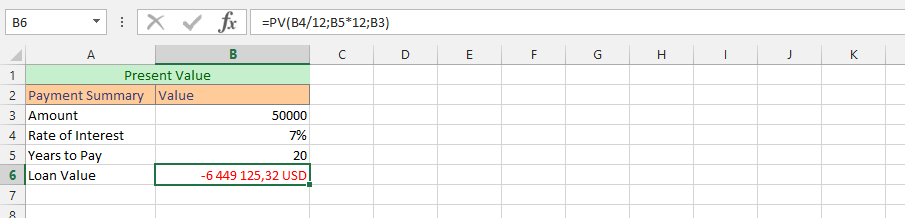### Complete PV Formula

This is the example, where we’d focus on finding the Present value for the business information that we have already layout. This is where the PV formula would be an extremely useful, and we are using the complete formula, which differentiates it from the rest. This example will provides us with the information that we’d need for the successfulness of acknowledging the present value, with an estimation we made for future value. This is the full information on what is pv.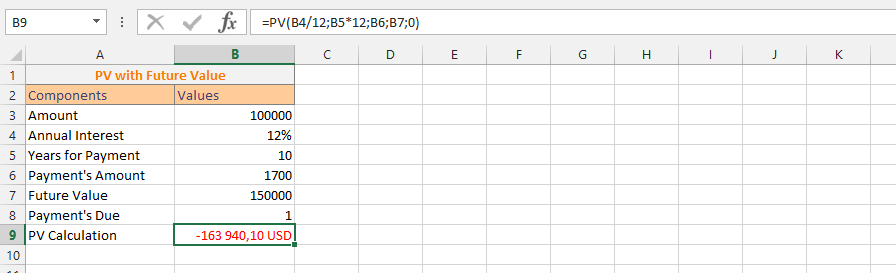### Present Value with Mathematical Symbol

This example specifically specifies that there needs to be a new deal, and we are assessing how it will be possible for the present value to have an impact under such circumstances about it. This is one of the places where the PV formula would be highly productive in the long run.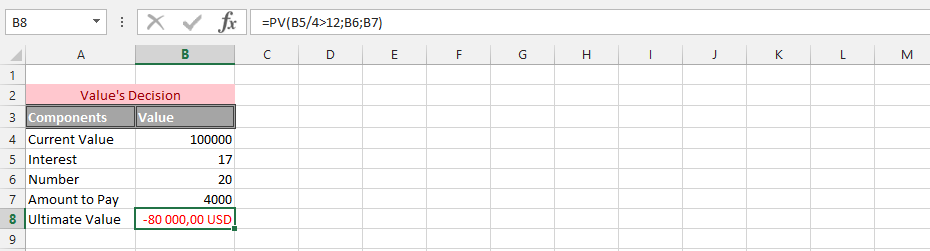### Evaluating Present Value with Additional Percentage

In this example, we have decided that we want to add some percentage to the present value. This is where the PV formula is extremely useful. This formula presented below includes the percentage that has been added to the formula.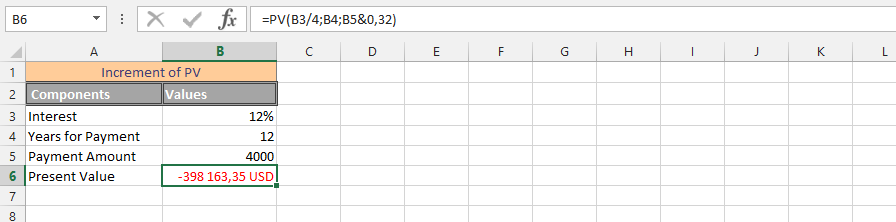### Using PV & AND Function Simultaneously

In this example, we want to find some answers that seem to have eluded us about the present value of payment. There have been estimations regarding how much the present value worth, and we need to know if that estimation is correct or not. This example is using the PV & AND functions simultaneously, and we have also estimated that the future value is 150000, so we need to know the present value, based on that.### Doubled Rate with Present Value

The present value needs to be estimated, with regards to the fact that we have estimated that the interest rates would be doubled at one point. The example clarifies that the possibility could happen in about three years, which is why we currently add it to the present value. For the successfulness of this calculation, we belief that the PV would be an excellent strategy for acknowledging this information. It is very easy to acknowledge this information, with the formula.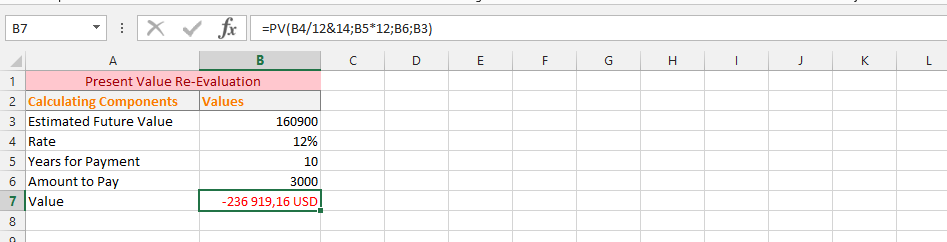### Acknowledging the PV with Text

The present value has been estimated, but we have rather used text than numbers in this case. There have been different clarifications that lead to us needing to use text, which is also possible with the PV (Present Value), and this possibilities have been productive in the formula that has been presented. We are now going to use the PV formula with text.### Present Value and OR Functions Simultaneously

There have been estimations that it is necessary for the present value to be flexible within the upcoming six months, and we would like to split the remaining payments for the upcoming months, but PV formula do not allow the possibilities of using 6 months, which makes creativity one fantastic way of solving this problem. Instead of using six months, as it does not agree to, we now use 6 years, and divide it by 6, as a solid solution to the current problem.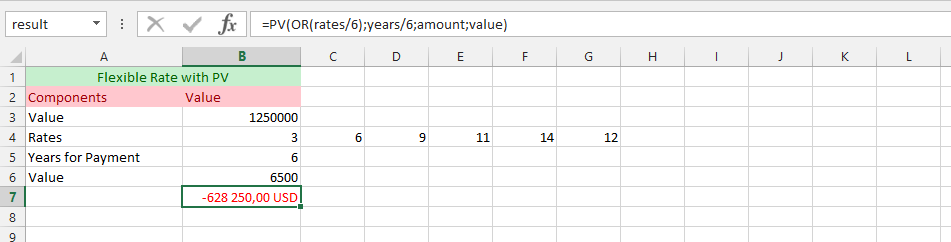### Just Numbers in Formula

The present values are being estimated with different formulas, so we are rather adding numbers than assigning the parameters with cells. This is where the example directly and sophisticatedly tackles the situation with regards to that estimations, as they have been outlaid.### PV Formula with another Spreadsheet

This example has been based on having the spreadsheet in one spreadsheet, and writing the formula in another spreadsheet.## Template

```Further reading: